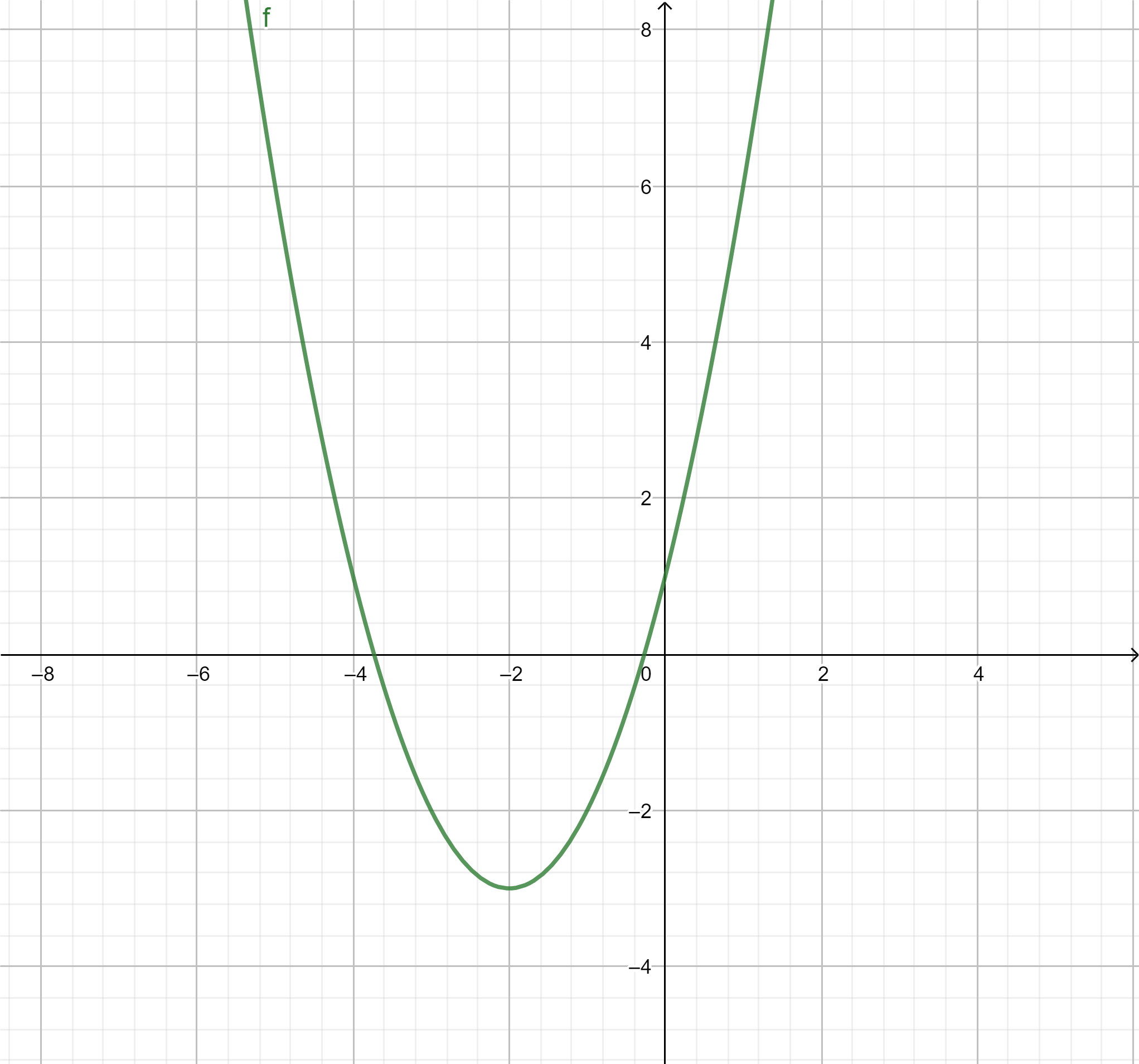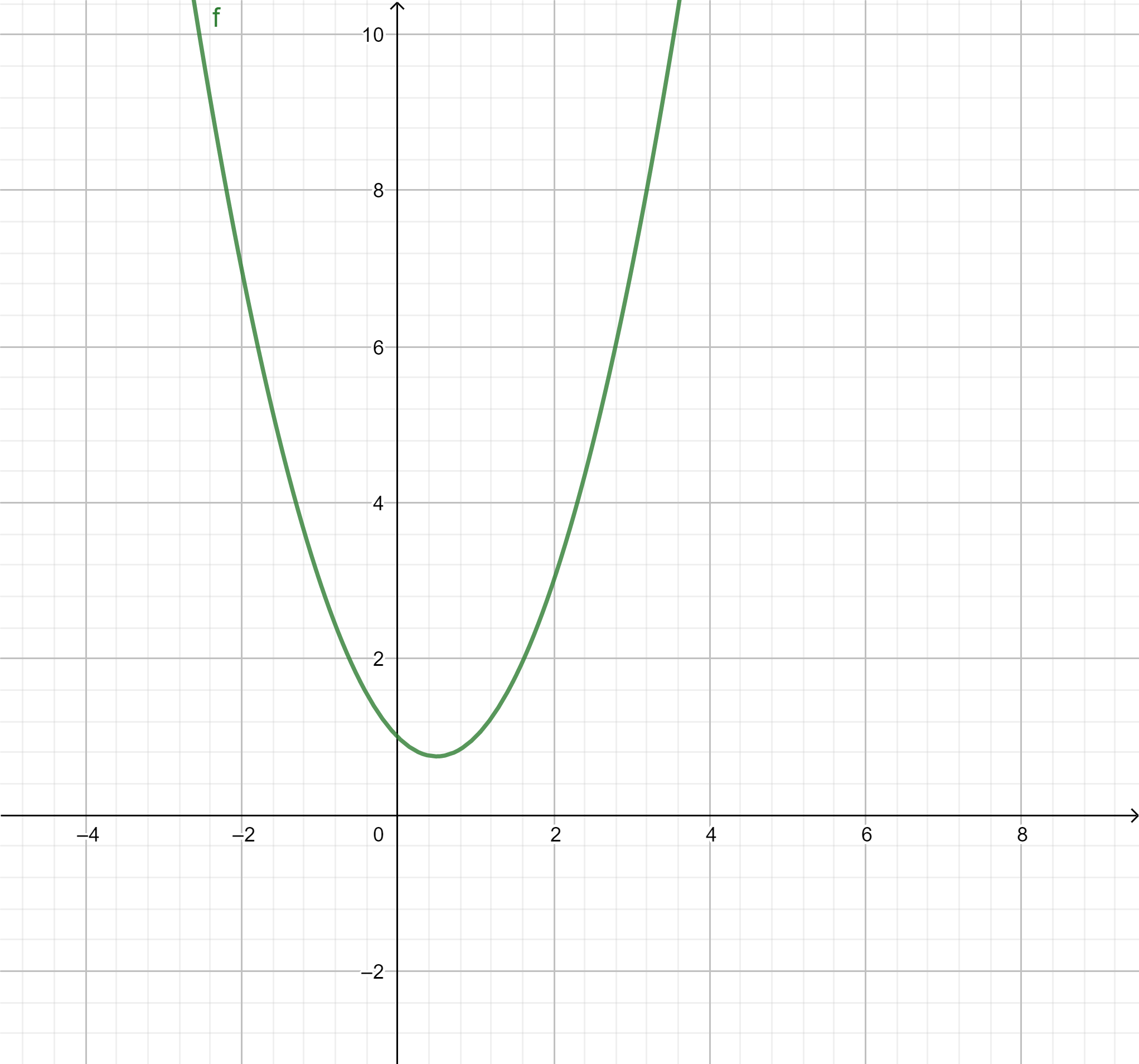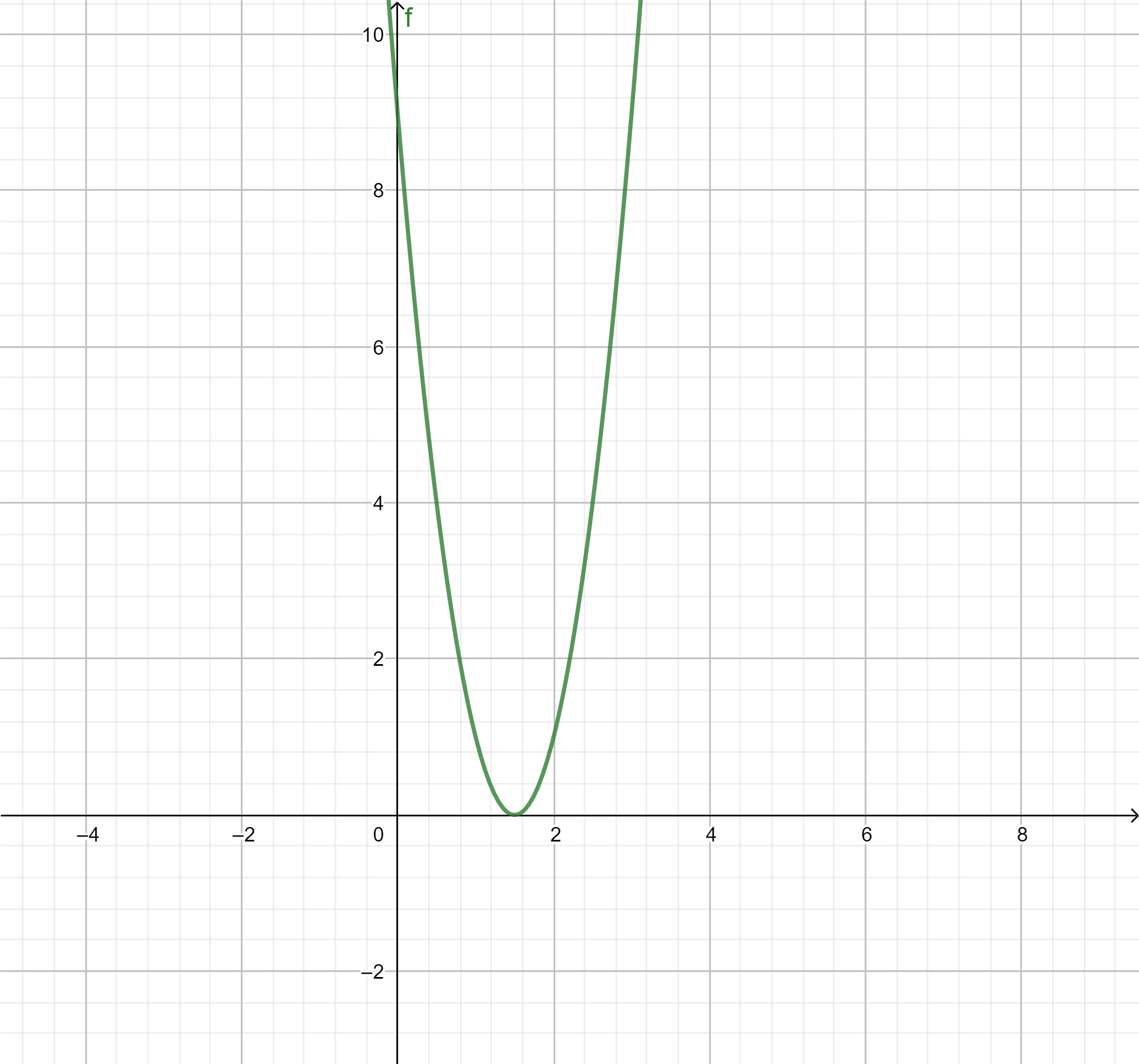QuestionAnswers

# If the Discriminant is zero for a Quadratic Equation, thenA. Graph will intersect the x-axis at two points.B. The graph will touch the x-axis.C. The graph will be above the x-axis.D. None of the above.

Hint: The nature of the roots of a quadratic equation can be determined from the value of the discriminant. The roots of an equation are the points at which the curve meets the x-axis. So, we can find the nature of the root and thus predict the graph.

We know that the nature of the roots of a quadratic equation can be found from its discriminant. If the discriminant is greater than zero, the equation will have two real and distinct roots. If the discriminant is zero, the equation will have a real root. If the discriminant is less than zero, the equation will have no real roots, it will have 2 complex roots.
Graphically, the roots of an equation can be defined as the points where the curve of the equation meets the x-axis.
So, if an equation has 2 roots, then the curve meets the x-axis at two points. Its graph is given by,If the equation has no real then the curve does not meet the x-axis and its graph is given by,And if the equation has only 1 root, the graph meets the x-axis at only one point. Its graph is given by,We are given that the quadratic equation has discriminant zero. So, the equation has only one root. So, the graph of the equation will touch the x-axis only once.
Therefore, the correct answer is option B.
Note: Discriminant of quadratic equation of the form $a{x^2} + bx + c = 0$ is given by $D = {b^2} - 4ac$.
If $D > 0$, the equation has 2 real and distinct roots. They are given by $x = \dfrac{{ - b \pm \sqrt {{b^2} - 4ac} }}{{2a}}$
If $D = 0$, the equation has a real root, which is given by, $x = \dfrac{{ - b}}{{2a}}$
If $D < 0$, the equation has complex roots.